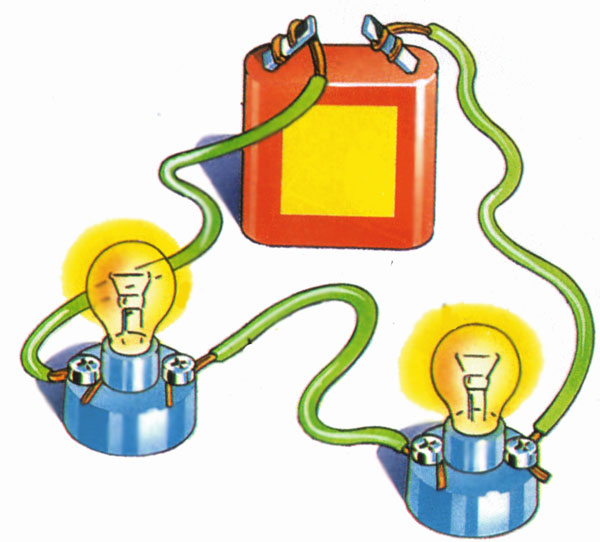What is Electronic Circuit?

An electronic circuit is a path, in which electric current can flow. A basic circuit is the combination of electrical components, which are wired together. The electronic circuit is made to do a certain job out of electricity.

Schematic

The drawing of an electric circuit is called schematic. It is used to simplify the electronic circuit so that we can understand how the components are connected together. All the electrical components are represented by their symbols, called schematic symbol.

Series Circuit

When the electronic components are connected in series with each other and power source, they are called to be connected in series. In a series connection, the components are connected in head-to-tail fashion. Consider the following example of a series circuit, in which resistors are connected in series.

In a series circuit the current in the all components is same, but the voltage is divided among them.

Parallel Circuit

A parallel circuit is the combination of electrical components in parallel with each other and the power source. In parallel circuits, components are connected in head-with-head and tail-with-tail fashion. Consider the following schematic of the parallel circuit, in which resistors are connected in parallel.

In a parallel circuit, the current is divided into each branch. But, the voltage of each branch is same.

Series-Parallel circuit

This circuit has both kinds of connections. Some components are connected in series and some components are connected in parallel. To deal with the series-parallel circuit, each branch of the circuit is considered separately for understanding the behavior of the circuit.

Consider the following circuit, in which the resistors are connected in series and parallel combination.

Ground of the Circuit

A ground is the reference point in a circuit. This point works as a return path for the electrical current back to the source. Every circuit has at least one ground. However, a circuit can have more than one ground. The voltage across each electronic component is measured with respect to ground.

The schematic symbol of the ground is: